## Appendix BFinite Fields

In this section, we study the finite fields. Such a field must have prime characteristic (the characteristic of a field is the smallest integer n such that 1 + 1 +1 (n times) is 0). Therefore, it contains one of the finite fields Fp. This makes it a finite dimensional vector space over Fp, so that its order must be some power of p. We will see that, up to isomorphism, there is exactly one field of a given prime power order. We will also show that choosing a non-trivial character of the additive group of a finite field gives an identification of this group with its Pontryagin dual, and we will study the Fourier transform in this context.

### B.1. Existence and uniqueness

We will show that for any power pk of p, there is a unique finite field of order pk, which is unique up to isomorphism1 . For convenience, write q = pk. Fix an algebraic closure Fp of Fp. Look at the set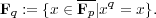Exercise B.1. If x,y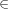Fq, then show that x + y and xy are in Fq.

It follows from the above exercise that Fq is a field (why?).

Exercise B.2. Let K be any field, and f(X)K[X] be of degree d. Show that f(X) can not have more than d roots in K.

Since the elements of S are roots of the polynomial Xq - X which has degree q, there can be no more than q of them.

Exercise B.3. Let K be any field. For a polynomial f(X)K[X]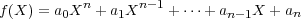define its (formal) derivative to be the polynomial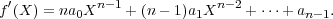Show that if a is a multiple root of f(X) (i.e., (X - a)2f(X)) then f(a) = 0.

The derivative of the polynomial Xq -X is the constant polynomial -1. Therefore, all its roots in Fp are distinct. This means that S has exactly q elements. Therefore there exists a subfield of order q in Fp. In particular there exists a finite field of order q.

On the other hand, in any field of order q, the multiplicative group of non-zero elements in the field has order q - 1. Therefore, each element of the field satisfies xq-1 = 1, or xq = x. Thus any subfield of Fq of order q must be equal to S.

Now any field of order q must have characteristic p, hence is an algebraic extension of Fp. Therefore, it is isomorphic to some subfield of Fp. We have seen that only such field is Fq. It follows that every field of order q is isomorphic to Fq. We have proved the following theorem:

Theorem B.4. For every power q of a prime number, there exists a finite field of order q, which is unique up to isomorphism.

### B.2. The multiplicative group of Fq

We present the proof of the following theorem straight out of Serre’s book [Ser73].

Theorem B.5. The multiplicative group Fq* is cyclic of order q-1.

Proof. If d is an integer 1, then let φ(d) denote the number of integers x with 1 x d such that (x,d) = 1. In other words, the image of x in Z∕dZ is a generator of Z∕dZ. The function φ(d) is called the Euler totient function.

Lemma B.6. If n 1 is an integer then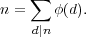Proof. If dn, let Cd denote the unique subgroup of order d in Z∕nZ, and Φd denote the generators of Cd. Then Z∕nZ is the disjoint union of the Φd. Φd had φ(d) elements. Adding up cardinalities, n = dnφ(d).

Lemma B.7. Let H be a finite group of order n. Suppose that, for all divisors d of n the set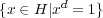has at most d elements. Then H is cyclic.

Proof. Let dn. If there exists xH of order d, the subgroup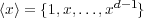is cyclic of order d. By hypothesis, every element y such that yd = 1 is in x. In particular, the elements of order d are the generators of x, and these are φ(d) in number. Hence the number of elements of order d is either 0 or φ(d). If it were zero for some dn, Lemma B.6 would show that the number of elements in H is strictly less than n, contrary to hypothesis. In particular, there exists an element of order n in H, and H so H is cyclic of order n.

To complete the proof of Theorem B.5, note that the equation xd = 1 is a polynomial equation, and hence, by Exercise B.2 has at most d solutions in Fq.

Corollary B.8. Every finite field is perfect.

### B.3. Galois theoretic properties

In general, if E is an extension of a field F, then every element xE can be thought of as an F-linear endomorphism of the F-vector space E, when it acts on E by multiplication. The trace of this map is denoted tr E∕F(x). The function trE∕F : E F is called the trace function of E over F. Likewise, the determinant of multiplication by x is denoted NE∕F (x). The function NE∕F : E F is called the norm map of E over F.

Since Fq2 is a quadratic extension of Fq, its Galois group is cyclic of order 2. Clearly, the map F : xxp is an automorphism of Fq2 that fixes Fq. Therefore, it must be the non-trivial element in the Galois group of Fq2 over Fq. F is called the Frobenius automorphism. In analogy with complex conjugation, we write F(x) = x for each xFq2.

Proposition B.9. Suppose xFq2. Then x = x if and only if xFq.

Let N and tr denote the norm and trace maps of Fq2 over Fq respectively. Then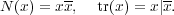Note that for any xFq2, N(x) = 0 if and only if x = 0.

Exercise B.10. Show that the norm map N : Fq2*Fq* is surjective. Conclude that for any xFq, the number of elements yFq2 such that N(y) = x is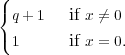### B.4. Identification with Pontryagin dual

Let ψ0 : Fq C* be a non-trivial additive character. Such a character is completely determined by its value at 1, which can be any pth root of unity different from 1. Then ψ : Fq C* defined by ψ(x) = ψ(trFqFp(x)) is a non-trivial additive character of Fq.

Proposition B.11. For each xFq, set ψx(x) = ψ(xx). Then xψx is an isomorphism from the additive group of Fq onto its Pontryagin dual.

Proof. The map xψx is clearly an injective homomorphism. By Proposition 1.2, it must also be onto.